# Inputs

## 1. Introduction

The total number of inputs is equal to 14.

Among these inputs, 5 are standard inputs and 9 are advanced inputs.

The aim is to define a list of working points characterized with the torque and the speed.

To know the framework in which the working points can be selected, the torque-speed envelope is computed considering the four inputs data: the maximum line current, the maximum line-line voltage, the command mode and the maximum speed to be considered.

By default, the list of working points is built with points on the torque-speed envelope.

But one can define a list of working points located anywhere under (or on) the torque-speed envelope.

Please refer to the section: Definition of the working point list.

## 2.1 Definition of the working point list

1. Overview

By default, the list of working points is automatically built by considering seven points on the machine torque-speed envelope. The torque-speed envelope depends on the four following input parameters:

• line current, rms
• Line-Line voltage, rms
• Command mode
• Maximum speed

It is also possible to define our own working point list by filling in a table with the targeted speeds and torques.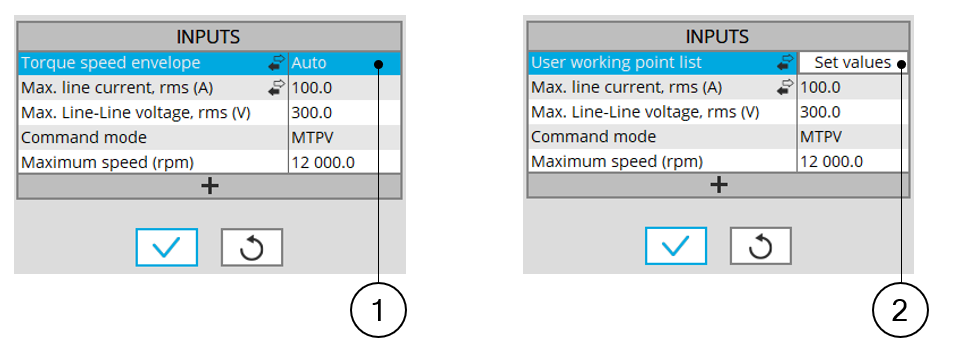Two ways for defining the working point list 1 Automatic mode: working points are automatically considered on the torque-speed envelope 2 We can define our own working point list by filling in a table with the targeted speeds and torques
2. Process to define the working point list

Two ways are possible to define the list of working points: either filling the table line per line or by importing an Excel file in which all the working points to be considered are defined.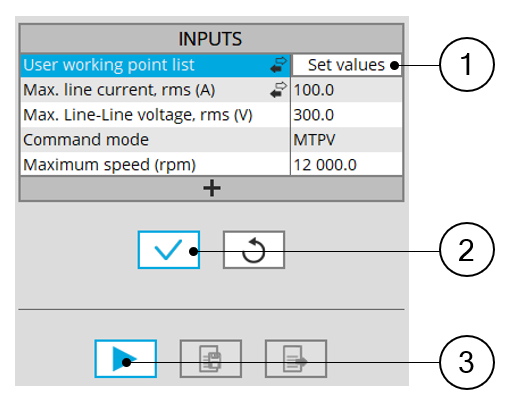List of working points to be defined 1 Click on the button “Set values” of the field“ User working point list” to define the working points in a dialog box. Refer to the next illustration which shows how to fill the working point table. 2 Button to validate the user input data 3 Button to run the computations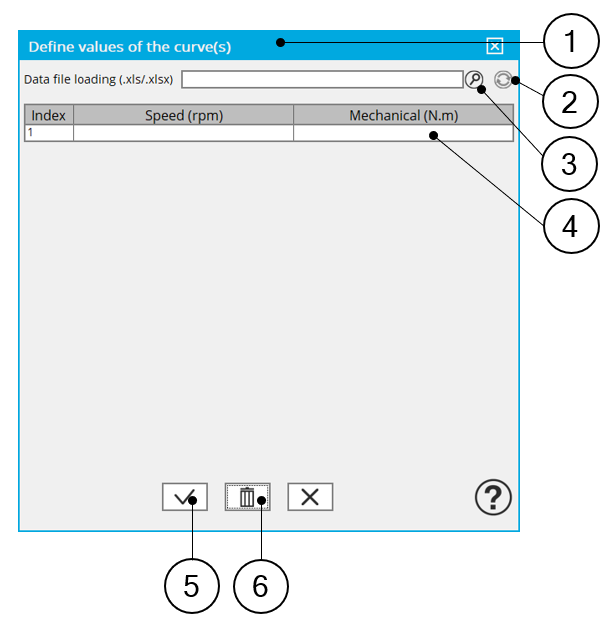Dialog box to define the list of working points 1 Dialog box opened after having clicked on the button “Set values” in the field “Cycle description” 2 Browse the folder to select an Excel file which is described the duty cycle 3 Button to refresh the table data when the considered Excel file has been modified 4 Fields to be filled with data to describe the duty cycle to be considered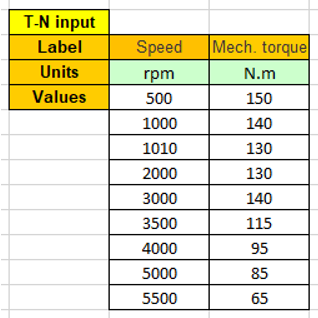Excel file template to define the list of working points with speed and torque data

## 2.2 Maximum line current, rms / Current density

There are two common ways to define the maximum line current.

Electrical current can be defined either by the current density in electric conductors or directly by indicating the value of the line current (the rms value is required).

When the choice of current definition mode is “current”, the rms value of the maximum line current supplied to the machine: “Max . l ine current, rms” ( Maximum line current, rms value ) must be provided.

When the choice of current definition mode is “density”, the rms value of the maximum current density in electric conductors “Max. Current density, rms” (Maximum current density in conductors, rms value) must be provided.

Note: The number of parallel paths and the winding connection are automatically considered in the results.

## 2.3 Maximum Line-Line voltage, rms

The rms value of the maximum line-line voltage supplying to the machine: “Max. Line-Line voltage, rms” ( Maximum Line-Line voltage, rms value ) must be provided.

Note: The number of parallel paths and the winding connection are automatically considered in the results

## 2.4 Command mode

For any applied command mode, the process consists of computing the torque-speed envelope.

Note: For additional information on this topic, please see the section dedicated to command modes (Performance mapping – efficiency map)

## 2.5 Maximum speed

The computation and analysis of the torque-speed curves are performed over a given speed range.

The maximum allowed value for the « Maximum speed » corresponds to 53000 rad/s - about 506000 rpm.

Note:
1. The maximum speed is considered build the following outputs:

• Radiated sound power spectrograms versus engine order or frequency
• Overall radiated sound power per engine order versus speed
• Overall weighted radiated sound power versus speed
2. As a function of the maximum speed value, following different cases must be considered:

Case 1 : The maximum speed is lower than the base speed N base (corner point speed of the torque-speed curve) N max < N base .

In that case, whatever is the command mode (MTPA or MTPV), the behavior of the machine will be studied over the speed range [0, N max ].

That allows the user to precisely choose the range of speed to be considered for computing and displaying the torque-speed curve and especially maps like efficiency map.

Case 2 : The maximum speed is greater than the base speed (corner point speed) N max > N base .

The relevance of the maximum speed given by the user is analyzed to evaluate if it is reachable by the machine.

If the user maximum speed is unreachable by the machine, the correction of this value is automatically performed.

The resulting new maximum speed is linked to a limit torque. This limit torque is obtained by applying a reduction coefficient to the base point torque.

3. For additional information on this topic, please see the section dedicated to command modes (Performance mapping – efficiency map)

## 3.1 Maximum engine order / No. Points per electrical period

Two kinds of inputs are possible:

Define the Max. engine order ( Maximum engine order ) or the No. points / elec. Period ( Number of points per electric period ).

When decomposing the Maxwell pressure, applied on the stator, to get its harmonic contributions, the “ max. engine order ” ( Maximum engine order ) is required to compute its decomposition in function of the time.

At a practical point of view, when the maximum engine order is equal to N, that leads to consider 2*N computation points over a complete rotation period of the rotor.

Note:
1. : The input "Engine order" is in connection with the frequency of vibration we want to be able to compute.

"Engine order" refers to a mechanical revolution period of the motor when frequency refers to the considered electrical period.

Obviously, both are linked with speed.

For instance, radiated sound power can be displayed either by considering frequency or engine order.

2. There are two possibilities, either set an engine order or a number of points per electrical period.

For transient computations the minimum needed number of points per electrical period is 40.

So, when the engine order is not high enough to reach this constraint It is automatically modified to get 40 computation points per electrical period.

## 3.2 Maximum mode / spatial order

The “ max. mode / spatial order ” ( Maximum mode / spatial order ) input allows the user to define the number of modes to be considered for the acoustic structural analysis. If the user selects 25, it means that the highest number of lobes in the stator deformation will be equal to 25 lobes. All deformations corresponding to more than 25 lobes will be dismissed.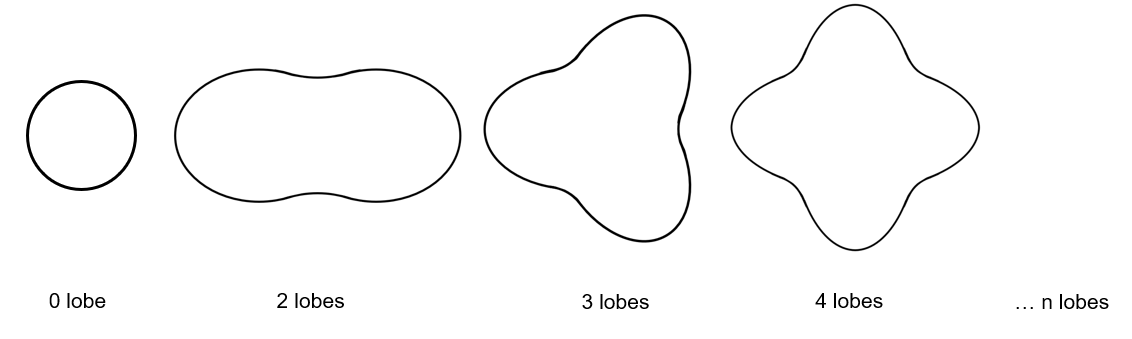Number of lobes of the stator mechanical structure

## 3.3 Number of computations per tooth pitch

The “ No. comp./tooth pitch ” ( Number of computations per tooth pitch ) allows to choose the number of Maxwell pressure evaluations per tooth. The more points selected, the more accurate the Maxwell pressure harmonic decomposition will be.

## 3.4 Number of points for speed interpolation

The “ No. points for speed interpolation ” (Number of points for speed interpolation) allows to manage the computation of the radiated sound power per engine order. It allows to manage the data interpolation between the speeds indicated as inputs. Thanks to that, the curves “Radiated sound power per engine order versus speed” and “weighted radiated sound power versus speed” can have a better discretization which leads to a better displaying of the local peaks.

The default value is equal to 100. The range of possible values is [50,300]

## 3.5 Number of computations for Jd,Jq

The “ No. comp. for Jd,Jq ” ( Number of computations for D-axis and Q-axis currents ) has the same definition than in the test “Performance mapping – Sine wave – Motor - Efficiency maps.

First, it is needed to compute the D-axis and Q-axis flux linkage in the J d - J q plane.

To get maps in the J d - J q plane, a grid is defined. The number of computation points along the d-axis and q-axis can be defined with the user input « No. Comp. for J d , J q » (Number of computations for D-axis and Q-axis currents) .

The default value is equal to 5. This default value provides a good compromise between accuracy and computation time. The minimum allowed value is 5.

## 3.6 Number of computations for speed

The “ No. comp. for speed ” ( Number of computations for speed ) has the same definition than in the test “Performance mapping – Sine wave – Motor - Efficiency maps.

The “No. comp. for speed” ( Number of computations for speed ) corresponds to the number of points to be considered in the speed range from 0 to the maximum speed.

Half of these points are distributed from 0 to the base speed. The remaining points are distributed from the base speed to the maximum speed.

In both cases, base speed is considered as an additional point.

Note: If the user input parameter “ No. comp. for speed ” is an odd number, one discretization point is automatically removed.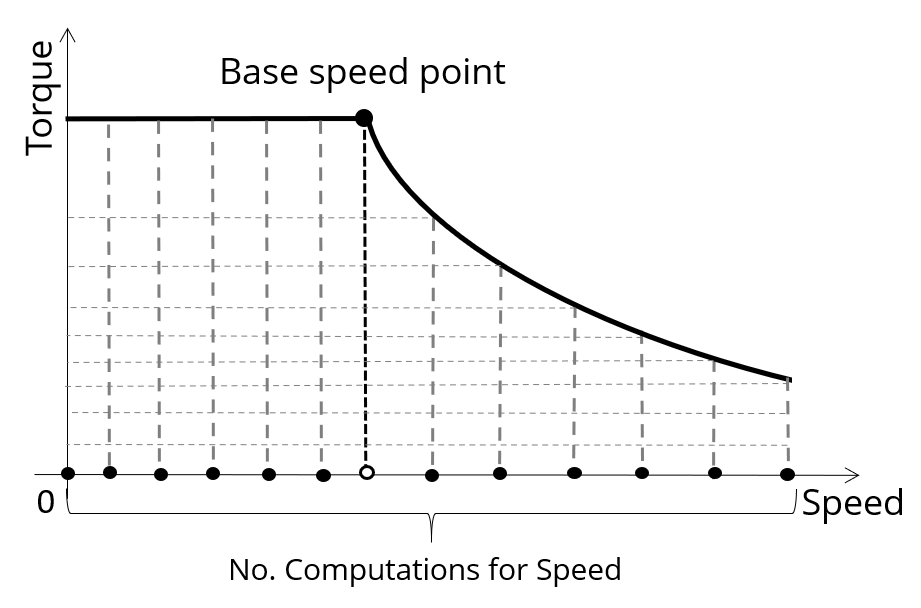Definition of the number of computations for speed

The default value is equal to 15, the minimum allowed value is 5. The maximum recommended value is 40.

Note: Increasing the number of computations can improve the convergence of the optimization used to define the torque-speed curve and the efficiency map. However, that also means longer computation time.

## 3.7 Number of computations for torque

The “ No. comp. for torque ” ( Number of computations for torque ) has the same definition than in the test “Performance mapping – Sine wave – Motor - Efficiency maps.

For the speed range [N base ; N max. ], the number of computations for torque is imposed by the number of computations for speed in the speed range [N base ; N max. ] (Red points in the image shown below).

The advanced user input parameter “ No. comp. for torque ” allows to finalize the grid within the torque range [0, T (N max. )] at the maximum speed (Black points in the image shown below).

The default value is equal to 7. The minimum allowed value is 3. The maximum recommended value is 20.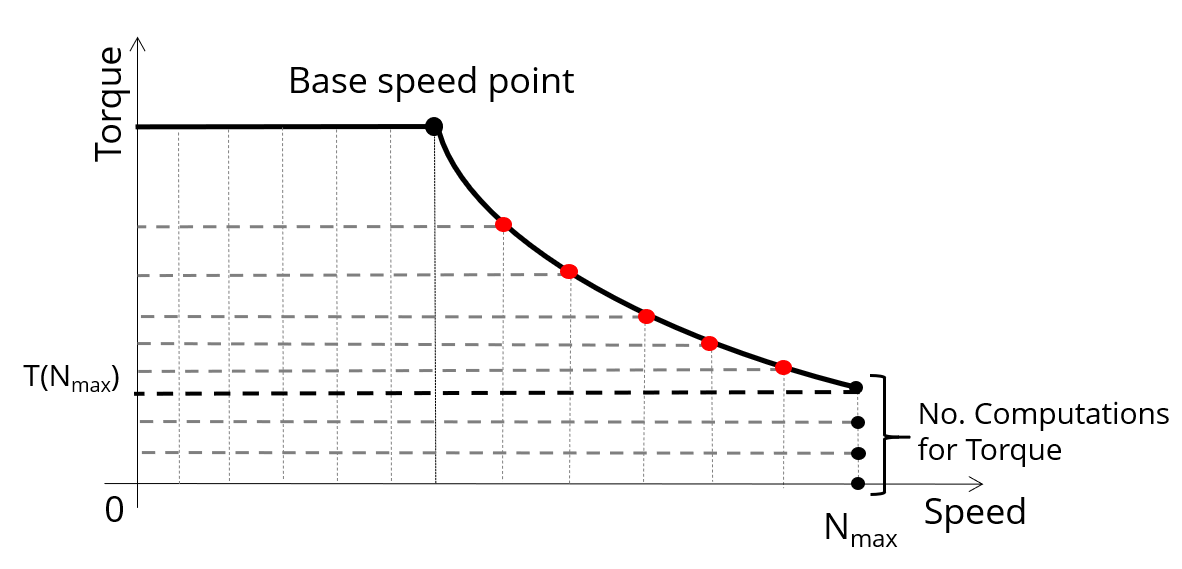Definition of the number of computations for torque – MTPV command mode

## 3.8 Mesh order

To get the results, the original computation is performed using a Finite Element Modeling.

Two levels of meshing can be considered for this finite element calculation: first order and second order.

This parameter influences the accuracy of results and the computation time.

By default, second order mesh is used.

## 3.9 Airgap mesh coefficient

The advanced user input “ Airgap mesh coefficient ” is a coefficient which adjusts the size of mesh elements inside the airgap. When the value of “ Airgap mesh coefficient ” decreases, the mesh elements get smaller, leading to a higher mesh density inside the airgap, increasing the computation accuracy.

The imposed Mesh Point (size of mesh elements touching points of the geometry) is described with the following parameters:

• MeshPoint = (airgap) x (airgap mesh coefficient)
• Airgap mesh coefficient is set to 1.5 by default.

The variation range of values for this parameter is [0.05; 2].

0.05 giving a very high mesh density and 2 giving a very coarse mesh density.

CAUTION:
Be aware, a very high mesh density does not always mean a better result quality.

However, this always leads to a huge number of nodes in the corresponding finite element model. So, it means a need of huge numerical memory and increases the computation time considerably.

## 3.10 Rotor d-axis location

The computations are performed by considering a relative angular position between rotor and stator.

For the reluctance synchronous machines, the rotor d-axis location is defined and automatically used to perform computations.

This value is characterized by the saliency topology. This is important to keep in mind this information it.

For more details, please refer to the document: MotorFactory_2022.2_SMRSM_IR_3PH_Test_Introduction – section “Rotor and stator relative position”.